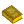## Numerical Derivative

While it is usually much easier to compute a Derivative instead of an Integral (which is a little strange, considering that more'' functions have integrals than derivatives), there are still many applications where derivatives need to be computed numerically. The simplest approach simply uses the definition of the Derivativefor some small numerical value of.Numerical Methods
Press, W. H.; Flannery, B. P.; Teukolsky, S. A.; and Vetterling, W. T. Numerical Derivatives.'' §5.7 in Numerical Recipes in FORTRAN: The Art of Scientific Computing, 2nd ed. Cambridge, England: Cambridge University Press, pp. 180-184, 1992.